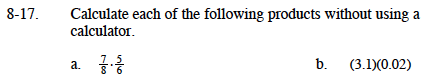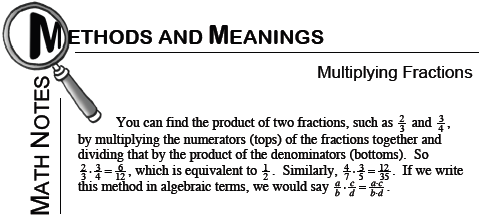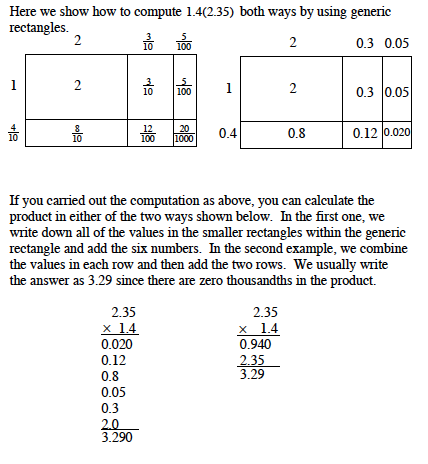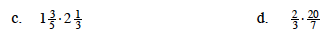### Home > MC1 > Chapter 8 > Lesson 8.1.1 > Problem8-17

8-17.Refer to the Math Notes box from Lesson 6.2.4 below for help.Refer to the Math Notes box from Lesson 6.3.1 below for help.Change the mixed numbers into fractions and then refer to part (a) for help.

$\frac{40}{21}=1\frac{19}{21}$